# 【Unity3D开发小游戏】2D光圈游戏

## 三、正文

### 1、摄像机设置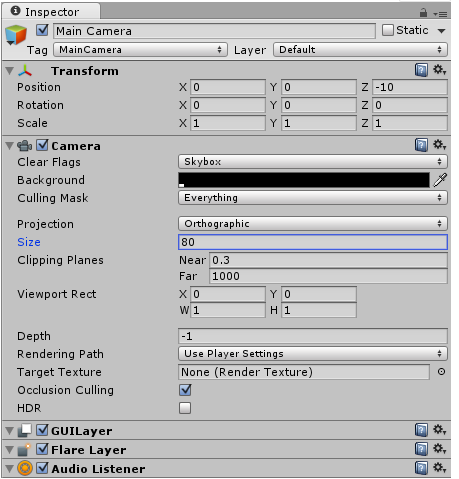### 2、灯饰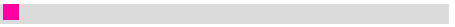所以我们最终得到了另一个预制件：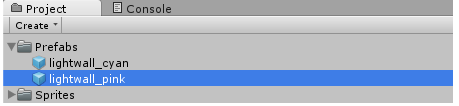### 3、玩家

Player添加物理组件：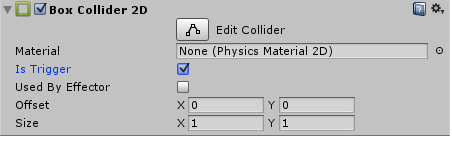注意：我们启用了IsTrigger以避免与玩家自己的“光环”发生碰撞。只要我们有IsTrigger启用后，玩家将只接收碰撞信息，而不会实际与任何东西发生冲突。这很快就有意义了。

``````using UnityEngine;
using System.Collections;

public class Move : MonoBehaviour {

// Use this for initialization
void Start () {

}

// Update is called once per frame
void Update () {

}
}
``````

``````using UnityEngine;
using System.Collections;

public class Move : MonoBehaviour {
// Movement keys (customizable in Inspector)
public KeyCode upKey;
public KeyCode downKey;
public KeyCode rightKey;
public KeyCode leftKey;

// Use this for initialization
void Start () {

}

// Update is called once per frame
void Update () {

}
}
``````

``````// Update is called once per frame
void Update () {
// Check for key presses
if (Input.GetKeyDown(upKey)) {
// Do stuff...
}
else if (Input.GetKeyDown(downKey)) {
// Do stuff...
}
else if (Input.GetKeyDown(rightKey)) {
// Do stuff...
}
else if (Input.GetKeyDown(leftKey)) {
// Do stuff...
}
}
``````

``````using UnityEngine;
using System.Collections;

public class Move : MonoBehaviour {
// Movement keys (customizable in Inspector)
public KeyCode upKey;
public KeyCode downKey;
public KeyCode rightKey;
public KeyCode leftKey;

// Movement Speed
public float speed = 16;

...
}
``````

``````// Update is called once per frame
void Update () {
// Check for key presses
if (Input.GetKeyDown(upKey)) {
GetComponent<Rigidbody2D>().velocity = Vector2.up * speed;
}
else if (Input.GetKeyDown(downKey)) {
GetComponent<Rigidbody2D>().velocity = -Vector2.up * speed;
}
else if (Input.GetKeyDown(rightKey)) {
GetComponent<Rigidbody2D>().velocity = Vector2.right * speed;
}
else if (Input.GetKeyDown(leftKey)) {
GetComponent<Rigidbody2D>().velocity = -Vector2.right * speed;
}
}

``````

``````// Use this for initialization
void Start () {
// Initial Velocity
GetComponent<Rigidbody2D>().velocity = Vector2.up * speed;
}
``````

``````public class Move : MonoBehaviour {
// Movement keys (customizable in Inspector)
public KeyCode upKey;
public KeyCode downKey;
public KeyCode rightKey;
public KeyCode leftKey;

// Movement Speed
public float speed = 16;

// Wall Prefab
public GameObject wallPrefab;

// Current Wall
Collider2D wall;

...
``````

``````void spawnWall() {
// Spawn a new Lightwall
GameObject g = (GameObject)Instantiate(wallPrefab, transform.position, Quaternion.identity);
wall = g.GetComponent<Collider2D>();
}
``````

``````// Update is called once per frame
void Update () {
// Check for key presses
if (Input.GetKeyDown(upKey)) {
GetComponent<Rigidbody2D>().velocity = Vector2.up * speed;
spawnWall();
}
else if (Input.GetKeyDown(downKey)) {
GetComponent<Rigidbody2D>().velocity = -Vector2.up * speed;
spawnWall();
}
else if (Input.GetKeyDown(rightKey)) {
GetComponent<Rigidbody2D>().velocity = Vector2.right * speed;
spawnWall();
}
else if (Input.GetKeyDown(leftKey)) {
GetComponent<Rigidbody2D>().velocity = -Vector2.right * speed;
spawnWall();
}
}
``````

``````// Use this for initialization
void Start () {
// Initial Velocity
GetComponent<Rigidbody2D>().velocity = Vector2.up * speed;
spawnWall();
}
``````

``````void fitColliderBetween(Collider2D co, Vector2 a, Vector2 b) {
// Calculate the Center Position
co.transform.position = a + (b - a) * 0.5f;

// Scale it (horizontally or vertically)
float dist = Vector2.Distance(a, b);
if (a.x != b.x)
co.transform.localScale = new Vector2(dist, 1);
else
co.transform.localScale = new Vector2(1, dist);
}
``````

``````public class Move : MonoBehaviour {
// Movement keys (customizable in Inspector)
public KeyCode upKey;
public KeyCode downKey;
public KeyCode rightKey;
public KeyCode leftKey;

// Movement Speed
public float speed = 16;

// Wall Prefab
public GameObject wallPrefab;

// Current Wall
Collider2D wall;

// Last Wall's End
Vector2 lastWallEnd;

...
``````

``````void spawnWall() {
// Save last wall's position
lastWallEnd = transform.position;

// Spawn a new Lightwall
GameObject g = (GameObject)Instantiate(wallPrefab, transform.position, Quaternion.identity);
wall = g.GetComponent<Collider2D>();
}
``````

``````// Update is called once per frame
void Update () {
// Check for key presses
if (Input.GetKeyDown(upKey)) {
GetComponent<Rigidbody2D>().velocity = Vector2.up * speed;
spawnWall();
}
else if (Input.GetKeyDown(downKey)) {
GetComponent<Rigidbody2D>().velocity = -Vector2.up * speed;
spawnWall();
}
else if (Input.GetKeyDown(rightKey)) {
GetComponent<Rigidbody2D>().velocity = Vector2.right * speed;
spawnWall();
}
else if (Input.GetKeyDown(leftKey)) {
GetComponent<Rigidbody2D>().velocity = -Vector2.right * speed;
spawnWall();
}

fitColliderBetween(wall, lastWallEnd, transform.position);
}
``````

``````void fitColliderBetween(Collider2D co, Vector2 a, Vector2 b) {
// Calculate the Center Position
co.transform.position = a + (b - a) * 0.5f;

// Scale it (horizontally or vertically)
float dist = Vector2.Distance(a, b);
if (a.x != b.x)
co.transform.localScale = new Vector2(dist + 1, 1);
else
co.transform.localScale = new Vector2(1, dist + 1);
}
``````

### 4、加入另一个玩家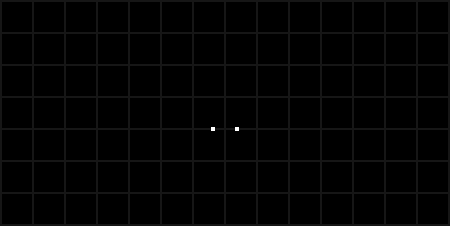### 5、碰撞检测

``````void OnTriggerEnter2D(Collider2D co) {
// Do Stuff...
}
``````

“Collider2D Co参数是玩家与之碰撞的对撞机。让我们确保这个对撞机不是玩家目前拖在身后的墙：

``````void OnTriggerEnter2D(Collider2D co) {
// Not the current wall?
if (co != wall) {
// Do Stuff...
}
}
``````

``````void OnTriggerEnter2D(Collider2D co) {
// Not the current wall?
if (co != wall) {
print("Player lost:" + name);
Destroy(gameObject);
}
}
``````

### 6、内容扩展

• 输赢屏幕
• 2名以上玩家
• 在线多人
• AI
• 一些特效
• 更好的Sprites等等 和往常一样，现在该由读者来让游戏变得有趣了。